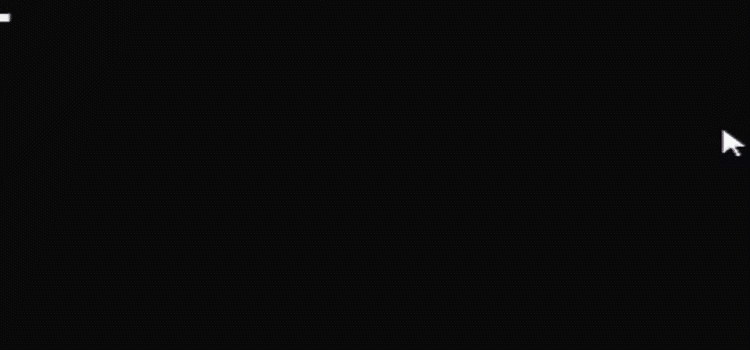﻿ C语言实现弹跳小球项目_C 语言_脚本之家

# C语言实现弹跳小球项目

更新时间：2022年05月12日 10:31:09   作者：辉小歌

## 一、项目描述和最终项目展示## 二、输出一个小球

```#include<stdio.h>
//静止的小球
int main(void)
{
int i,j;
for(i=0;i<6;i++)
printf("\n");
for(j=0;j<6;j++)
printf(" ");
printf("①\n");
return 0;
}```## 三、下落的小球

```//下降的小球
#include<stdio.h>
#include<windows.h>
int main(void)
{
int i,j;
int x,y;
int sum=0;
x=10;
y=10;
for(x=1;x<10;x++)//控制行数，即下落的距离
{
system("cls");
sum=0;
for(i=0;i<x;i++)//输出行数
{
printf("\n");
sum++;
}
for(j=0;j<y;j++)//距离原点的横着的距离
{
printf(" ");
}
printf("①\n");
}
return 0;
}```## 四、来回弹跳的小球

```//上下弹跳的小球
#include<stdio.h>
#include<stdlib.h>
int main(void)
{
int i,j;
int x=0;
int y=10;

int height=20;//最大高度
int velocity=1;//你的加速度
while(1)
{
x=x+velocity;//高度是越来越高
system("cls");

//输出小球前的空行
for(i=0;i<x;i++)
{
printf("\n");
}
for(j=0;j<y;j++)
{
printf(" ");
}
printf("①");
printf("\n");
if( x == height )//到底的时候，加速度变为负数
velocity = -velocity;
if( x == 0 )//到顶的时候，加速度变为正数
velocity = -velocity;
}
}```## 五、最终项目实现

```#include<stdio.h>
#include<stdlib.h>
int main(void)
{
int i,j;
int x=0;
int y=0;

int velocity_x=1;//x加速度
int velocity_y=1;//y加速度

int left=0;//左边的边
int right=20;//右边的边
int top=0;//顶部
int bottom=10;//底部

while(1)
{
x = x+velocity_x;
y = y+velocity_y;

system("cls");
for(i=0;i<x;i++)
{
printf("\n");
}
for(j=0;j<y;j++)
{
printf(" ");
}
printf("①");
printf("\n");

if( (x==top) || (x==bottom) )
//加速度正负的变化
{
velocity_x=-velocity_x;
}
if( (y==left) || (y==right) )
{
velocity_y=-velocity_y;
}
}
}```